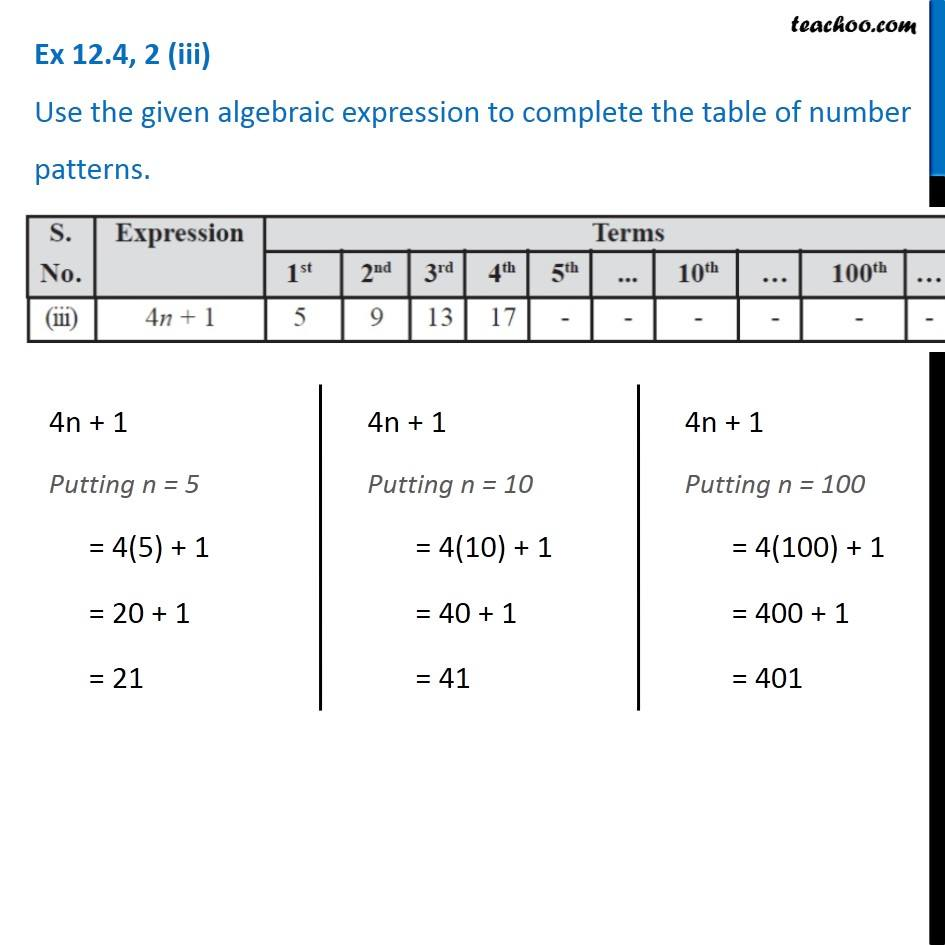Observing patterns

Chapter 10 Class 7 Algebraic Expressions
Serial order wiseLearn in your speed, with individual attention - Teachoo Maths 1-on-1 Class

### Transcript

Question 2 (iii) Use the given algebraic expression to complete the table of number patterns. 4n + 1 Putting n = 5 = 4(5) + 1 = 20 + 1 = 21 4n + 1 Putting n = 10 = 4(10) + 1 = 40 + 1 = 41 4n + 1 Putting n = 100 = 4(100) + 1 = 400 + 1 = 401